Journal of Statistics Education, V8N3:McLean

# The Predictive Approach to Teaching Statistics

Alan McLean
Monash University

Journal of Statistics Education v.8, n.3 (2000)

Copyright (c) 2000 by Alan McLean, all rights reserved. This text may be freely shared among individuals, but it may not be republished in any medium without express written consent from the author and advance notification of the editor.

Key Words: Prediction; Prediction interval; Probability model.

## Abstract

Statistics is commonly taught as a set of techniques to aid in decision making, by extracting information from data. It is argued here that the underlying purpose, often implicit rather than explicit, of every statistical analysis is to establish one or more probability models that can be used to predict values of one or more variables. Such a model constitutes 'information' only in the sense, and to the extent, that it provides predictions of sufficient quality to be useful for decision making. The quality of the decision making is determined by the quality of the predictions, and hence by that of the models used.

Using natural criteria, the 'best predictions' for nominal and numeric variables are, respectively, the mode and mean. For a nominal variable, the quality of a prediction is measured by the probability of error. For a numeric variable, it is specified using a prediction interval. Presenting statistical analysis in this way provides students with a clearer understanding of what a statistical analysis is, and its role in decision making.

# 1. Introduction

1 The typical introductory text in business statistics claims, in one way or another, that the use of statistics is to aid in decision making in conditions of uncertainty. This is true, but few texts do much, in any general way, to show how statistical analysis does aid in decision making. Yet there is an underlying structure to the use of statistics that provides a unifying theme for the subject, but which is rarely made apparent to students. This underlying theme is that the use of statistics is always in prediction.

2 Here is a selection of quotes from the introductory chapters of some well-known texts:

Statistics is a body of principles and methods concerned with extracting useful information from a set of numerical data to help managers make decisions. (Selvanathan 1994, p. 3)

Statistical thinking can be defined as thought processes that focus on ways to understand, manage and reduce variation. (Levine 1997, p. 4)

Statistics is a body of concepts and methods used to collect and interpret data concerning a particular area of investigation and to draw conclusions in situations where uncertainty and variation are present. (Bhattacharyya and Johnson 1977, p. 1)

Mathematical statistics is the study of how to deal with data by means of probability models.... In its broadest sense, statistical methods are often described as methods for making decisions in the face of uncertainty. The outcome of an experiment is usually uncertain but, hopefully, if it is repeated a number of times one may be able to construct a probability model for it and make decisions concerning the experimental process by means of it. (Hoel 1971, p. 1)

From the behavioural scientist's perspective, statistics are tools that can be used to unravel the mysteries of data collected in a research study. In particular, they allow the researcher to summarize the data and to distinguish between chance and systematic effects. (Shavelson 1981, p. 1)

3 As can be seen, statistics is seen variously as being concerned with controlling variability, making decisions, drawing conclusions, extracting information from data, and distinguishing real from chance effects. These views are, of course, not unrelated, but they do indicate the variety of approaches to teaching the subject. To complicate matters, most texts present a variety of views with different topics, resulting in a fragmented approach. Many textbooks concentrate on cross-sectional data and emphasise the distinction between descriptive and inferential statistics. Some texts (for example, Levine 1997) refer to Deming's (1950) distinction between enumerative and analytical studies. Texts written for business students typically provide a chapter or two on time series, but those chapters usually seem to be thematically isolated from the main text.

4 Two things can certainly be said about statistics. First, it is a practical discipline. It provides mathematical tools to use in practical situations, a set of techniques to be applied to the real world, in the same way that mathematics does. As with mathematics, statistics can be studied for its theoretical principles alone, but to most people it is only in applications that it is of value.

5 Second, it is intimately concerned with probability theory. Descriptive statistics can be taught without reference to probability, and there are applications of probability theory, such as quantum theory, that are not normally considered as part of statistics. But generally statistics can reasonably be considered to be applied probability. To some this may seem a contradiction: probability appears to be abstract and far from practical. In fact, however, probability is (to quote Laplace) merely "common sense reduced to calculus" (Laplace 1814, p. 196).

6 The view expressed in this paper is that statistics in action is always concerned with making decisions. These decisions are based on using probability to model the real world in order to predict what is likely to happen under various scenarios, and data are used to select, establish, and validate the models used. The components of this predictive approach are therefore the concept of a probability model, use of descriptive statistics to establish the model to be used, use of the probability model to make predictions, and use of predictions to make decisions. By adopting this view, we can have a unified approach to teaching statistics, with obvious benefits to students.

7 One finds fragments of this predictive approach in textbooks, particularly in introductory regression, where the need for prediction is used to motivate the development, and there is some emphasis on modelling. Many texts (for example, Levine 1997, p. 579) introduce the concept of a prediction interval for values of the response variable, albeit with little or no explanation of the concept. It is not pointed out that this concept is also appropriate in a univariate analysis.

8 In teaching regression there is usually some emphasis on prediction. It is recognised that the reason for carrying out a regression analysis is to predict the dependent variable. On the other hand, analysis of variance and cross tabulation are seen as extensions of basic statistics, in which subpopulations are compared in terms of a numeric or nominal variable, respectively, and the predictive usage is ignored. But the aim in each of these analyses is to identify a relationship that can provide better forecasts than are possible without it. Prediction is again the underlying purpose behind the analysis.

9 I proceed to discuss the components of the predictive approach, starting with the key concept of a probability model. This will be familiar to all working statisticians, but it is rarely emphasised in introductory courses. A key idea in the predictive approach is that it should be made clear to students from the start that the use of probability involves probability models.

# 2. Basic Vocabulary

10 Many of the learning problems of students originate from an inadequate knowledge of the basic vocabulary, reflecting a lack of understanding of the concepts encapsulated in the words. The terms used vary, but the important ones are briefly as follows.

11 A set of data may be collected as cross-sectional data (or snapshot data), describing a set of entities at a point in time, or it may be collected as one or more time series, describing a single entity over time, or as a longitudinal study (or panel data), describing a set of entities over time. The set of entities forms a population, or a subset of a population, called a sample. The data comprise values of one or more variables, each of which describes some characteristic of the entities. (Most of the real problems with the use of statistics arise because of the distinction between such characteristics and the variables used to measure them.) In the case of time series data, the variation is over time; for cross-sectional data it is over the members of the population; for panel data it is over both time and entities. Finally, the variables are distinguished according to their nature. The most useful classification is to identify a variable as nominal (the values are simply labels), ordinal (the values have some order), or numeric (the values have both order and scale).

12 This terminology applies whether discussing probability or statistical data. Probability is concerned with future values of the variables; with data the values have been recorded. In terms of probability, for each variable the 'next' value is uncertain, so the variable is called a random variable. In the case of time series, this is because the next value is still in the future, still to be generated by Nature. For cross-sectional data, it is because the next value is to be measured on an entity that is yet to be selected. It is clear that the means by which the entity is to be selected is of crucial importance in the nature of the variation.

# 3. Probability

## 3.1 Probability Models

13 Probability is commonly introduced as being concerned with an action whose outcome is uncertain. If the set of all possible outcomes is clearly identified, the action is sometimes called an experiment. In applications it is necessary to decide what outcomes are to be admitted as 'possible.' For example, in tossing a coin we would exclude such results as the coin landing on an edge, or being hijacked by a passing bird.

14 In terms of the basic vocabulary above, observing the outcome of an experiment is synonymous with measuring a random variable. (Some authors restrict the term 'random variable' to the case where it is numeric, in which case this observation needs to be modified. This seems to be an unnecessary complication.) A numeric random variable may be treated as discrete or continuous.

15 To each possible outcome considered -- equivalently, each possible value of the random variable -- is assigned a probability, by giving a numerical value or by formula, to give a probability distribution. This probability distribution, in practice, is always a model of the real world, not a simple description of it.

16 It can be argued that in the 'real' world, though an event may be said to be uncertain, there is actually no such thing as an 'uncertain event.' The result of an action is unpredictable because the set of processes involved is simply too complex. There is a complex deterministic process underlying the action, which the statistician replaces by a manageable probabilistic model. In the archetypal example, tossing a coin, if one knew everything about how the coin was held and at what angle, how hard it was flipped and in what direction the thumb moved, and the distribution of density within the coin, then the result of the toss could be predicted. For practical purposes, this computation is impossible. (See, for example, DeGroot 1986). Uncertainty exists because the processes involved are so complex that they cannot be known. To our perception the result is unpredictable, so we call it 'chance.' In other words, even at the intuitive level, chance is a model that simplifies the underlying real process.

17 On the other hand, it can also be argued that for an individual, the 'real world' is what is perceived by that individual, so in this sense chance exists in the real world. Putting aside such philosophical questions, probability as used is always in the form of a model of the world. A full statement of the model includes specification of the outcomes to be considered, definition of the measurement process, possibly identification of a variable as continuous (since recorded values must be discrete), and so on. It also involves the specification of the probabilities used, and other assumptions such as independence.

18 In teaching, the concept of a probability model becomes more explicit when the standard distributions are introduced. Students learn the conditions, for example, under which a binomial model may be applicable. It is important that students learn that the binomial is only a model that sometimes describes a real world situation quite well. The normal model is typically motivated by statements like 'experience shows that this type of variable has (approximately) a normal distribution...' Students, when they ask about behaviour in the tails, may force the response that normality 'is a good model near the centre of the distribution.'

## 3.2 Probability and Prediction

19 Bearing in mind the proposed emphasis on practical application in teaching statistics, two points should be made about probability. First, the role of probability is to enable predictions about the likely results of a specified action to be made. The word 'predict' is used here in the sense of specifying the probable result, with an estimated probability of its occurrence when the specified action is carried out.

20 In the case of time series, where variation is over time, and this generates the uncertainty in future values, this usage is clear, and is generally fairly clearly made in textbooks. For cross-sectional data, where the variation is over the members of the population, and the uncertainty arises from the selection process, it is perhaps less obvious. In this case, the role of probability is to make a statement about the likely result of a future random selection -- that is, to predict the result of such a selection.

21 People use probability models to predict what will happen in everyday decision problems. 'If I run across the road now, I am likely to be knocked over!' Stereotypes of people are probability models -- 'If this man is of ethnic origin X, he is probably stupid/ talkative/ a poor driver.'

22 In everyday life, these models are intuitive, based very much on personal experience and, often, personal prejudice. In more formal decision making, they may be more objective. The fundamental difference is that statistical methods can be used to test if a particular model is applicable, and to choose the best model from a number of possible models. One way of thinking about statistics is as a set of techniques to help people to 'learn by experience.'

## 3.3 What 'Is' Probability?

23 The view of probability described here is probably closest to the operational subjectivist view of de Finetti (1972). A statement of probability is a statement about an individual action. The probabilities are based on experience, which might take the form of noting how often each outcome has occurred in the past, but which must always be processed in generating the model. The proportion of times result X occurs in a sequence of (independent) repetitions of that action is a different thing from its probability of occurrence on a single trial, although it is perfectly reasonable, under suitable conditions, to use the one to provide a measure of the other. In life insurance, for example, measuring a person's probability of death in the coming year by using the historical proportion of people of the same age, with similar demographics, who die in the following year is very sensible. But to say that the probability for that person is the proportion is to confuse two different things. In life insurance, of course, the important thing from the company's viewpoint is the proportion of deaths, not what happens to an individual, so there is some excuse for making this confusion.

24 The predictive model approach is consistent with the Bayesian approach, which is primarily concerned with the concept of revising a prior probability model after obtaining sample data, giving a posterior model. It does not necessarily require that the prior model be subjective, though the approach seems to be often described in this way.

## 3.4 Usefulness of a Model

25 A key idea in the predictive approach is that a particular probability model should only be used if it works, preferably if it works better than alternative models. It 'works' if it produces good predictions that lead to successful decisions. The success of decisions can in the long run only be assessed through experience.

## 3.5 Probability With Cross-Sectional Data

26 For cross-sectional data, a variable is measured on a population, and the 'probability distribution of the variable' refers to the probability of each value when a member of the population is selected randomly. If a variable has been measured for all members of a population, the probability distribution is known, and this can be used, perhaps with some simplification through grouping of data, as an 'empirical model.' Alternatively, the empirical model can be approximated by some standard model. Conceptually the empirical model is simpler, but it is likely to be computationally more intensive.

27 Because the notion of probability enters through the selection of the individual rather than through the passage of time, probability questions can be posed and answered in terms of expected proportions. This has advantages -- most people find it easier to work in terms of the more concrete 'proportions' than the more abstract 'probabilities.' Importantly, everything said here about the use of a model applies whether it is phrased in terms of probabilities or expected proportions.

28 The way the question is asked, when expressed in terms of proportions, depends on the case, particularly on the nature of the population. For example, suppose an airline spaces its economy class seating so that people less than 1.83 metres tall are comfortable. What is the probability that a randomly selected traveller will be comfortable? In this case, not all the population of potential economy class travellers will actually travel, so the question can be asked as: What proportion of travellers are expected to be comfortable? but not as: What proportion of travellers will be comfortable?

29 On the other hand, if the probability question is: What is the probability that a randomly selected person will be less than 1.83 cm tall? the question can be asked as: What proportion of people will be less than 1.83 cm tall?

# 4. Prediction

30 The goal in the previous section was to argue that probability is used in the form of probability models which are used to make predictions, in the sense of specifying likely outcomes and their associated probabilities. The next step is to develop the idea of prediction.

31 First, note that models in practice are frequently incompletely specified. This is particularly true for the everyday decisions that we make all the time. In crossing a road, for example, when the traffic is heavy, the model used is quite imprecise: 'The probability of getting across safely is small.' It is nevertheless a genuine probability model on which a decision is based. In formal statistical argument it is frequently sufficient to use a model that is incompletely specified. For example, in short term forecasting of demand, it may be sufficient to use a model with constant mean and random fluctuations, and simply smooth the data using exponential smoothing. In this context a 'model' is not necessarily a fully specified model.

## 4.1 The Best Prediction for a Nominal Variable

32 Recognising that a probability distribution is used to predict what will happen when an experiment is carried out, what is the 'best' prediction? This of course depends on the criterion used, which in turn depends on the type of variable. Freeman (1965) and Foddy (1988) introduce the idea of an average as a 'best guess.'

33 For a nominal variable, it is reasonable to minimise the probability of error, and define the best prediction as the outcome that is most likely to eventuate, that is, the mode. This can also be described as the maximum likelihood prediction.

34 This use of the mode has nothing to do with 'centrality' -- in any case this concept is meaningless with a nominal variable -- and there may not be a single 'best prediction.' It is necessary to know the probability of each outcome in order to determine the mode. For a cross-sectional variable, under random sampling, this is the proportion of the population for each value of the variable.

35 How good is the best prediction? Using this criterion, the quality of the forecast is specified by giving the probability of its being correct. This is for most people a meaningful way of expressing the result.

36 Suppose I have a model for predicting tomorrow's weather:

 Weather Probability Rain most of the day 0.20 Rain periods and cloudy 0.15 Occasional showers, sunny periods 0.18 Overcast, dry 0.25 Fine and clear 0.22

For present purposes it does not matter whether this is a purely personal guesswork model, or the net result of a full scale computer model. Based on this model, the best prediction is that it will be overcast and dry, with a 25% chance of being correct.

37 In this example the prediction is not very good, with only a 25% chance of being correct, although it is the best available. Waiting till tomorrow will tell whether or not the prediction was correct, but it will not tell whether or not the model is a good one. Note that it is meaningless to ask if the model is 'true'; one may ask only if the model is 'useful.'

38 The quality of a model can only be assessed from experience. In some circumstances, such as tossing a coin, the same model is used a number of times, so the quality of the model can be assessed by comparing the model with the distribution of results. In other circumstances, such as betting on a horse race, the model is applied only once, so its quality can be assessed only by the track record, as a generator of good models, of the person generating this particular model. This is the root of the difference between frequentist and subjectivist notions of probability.

## 4.2 The Best Prediction for a Numeric Variable

39 With a numeric variable, if the number of different outcomes is small, it is again reasonable to minimise the probability of error, so that the best prediction is the mode. If the variable is modelled as being continuous, this maximum likelihood predictor is obtained by differentiating the density function with respect to the value of the variable. (This contrasts with maximum likelihood estimation in that in maximum likelihood estimation the values of the variable are known while the parameters are not, so differentiation is with respect to the parameters, while here the parameters are known.) This does not give the most probable value of the variable, since the probability of an individual value is not defined for continuous variables, but it does give a prediction close to which the observed value is highly likely to lie. That is, the prediction error is likely to be small. (The situation is rather more complex than indicated here. For example, if the distribution has no stationary point, as in the exponential distribution, the maximum is not found by differentiation but is simply a boundary value. If the distribution is multimodal, the global maximum may not give the required good prediction. It can be argued that in this case a better model can be obtained that identifies the distribution as a mixture of simple unimodal distributions. In any case, the objective here is to indicate the relationship of the approach to that of likelihood.)

40 The numeric scale gives the option of using this concept of prediction error, then choosing the best forecast as that which in some way minimises the likely error. The almost invariable choice is to minimise both the absolute expected error and the expected squared error. This is achieved by using the mean as the predictor. Then the absolute expected error is zero, ensuring that the prediction has no bias built in, and the expected squared error is just the variance. If in comparing predictions across variables, models, or populations, the mean is used in each case, the predictions will have zero expected error. The best prediction will then be the one with the smallest variance. Call this the least squares predictor.

41 For a normal model, the maximum likelihood predictor (the mode) and least squares predictor (the mean) are the same. This echoes the fact that for a normal model the maximum likelihood and least squares estimators are the same.

42 A different function of the error, or loss function, can be used as the criterion of likely error, leading in general to a different 'best predictor.' For example, using the expected absolute error leads to the median being preferred.

## 4.3 Prediction Intervals

43 For a numeric variable, assuming the mean is used for prediction, the absolute expected error is zero, so forecast quality is measured by the variance. This is generally not meaningful in practical terms, in contrast to the nominal case, where the forecast quality is measured by the probability of error. A more practically meaningful measure is to use a prediction interval -- an interval in which the result will lie with specified probability.

44 Prediction intervals can be calculated for any distribution, but this is rarely done in the textbooks. Most elementary texts provide exercises involving the calculations for the normal distribution, but the concept of a prediction interval is not developed. For a normally distributed variable, a symmetric two-sided (1-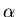)% prediction interval, for example, has limits.

45 For a time series, the meaning of a prediction interval is -- in terms of viewing a probability statement as about the future -- immediately clear. If daily demand for a product is modelled as independent of demand on previous days and normally distributed with mean 200 and standard deviation 20, the predicted demand for tomorrow is 200. A symmetric 95% prediction interval on this forecast has limits

200 ± 1.960 × 20 = 200 ± 39 = 161 to 239.

46 If the model is a good description of reality, that is, if it is 'valid,' tomorrow's demand will be within this interval with probability approximately 0.95. Conversely, there is a 5% chance that the error in prediction will be greater than 39. (This is only meaningful if one postulates the existence of some supermodel of the demand that describes 'reality' perfectly, and the chosen model approximates this. How one interprets the probability 0.95 depends on whether one is a frequentist or Bayesian.)

47 For a cross-sectional variable a prediction interval -- again in terms of viewing a probability statement as about the future -- is as follows. If the heights of a population are modelled as normally distributed with mean 170 cm and standard deviation 10 cm, the height of a randomly chosen member of the population is predicted to be 170 cm. A symmetric 95% prediction interval on this forecast has limits

170 ± 1.960 × 10 = 170 ± 20 = 150 to 190.

48 If the model is valid, there is a 95% chance that a randomly chosen person's height will be in this range. If the model is described in terms of expected proportions, the interval means that 95% of the population have heights in this range. It must be emphasised that a prediction interval is only meaningful to the extent that the model on which it is based accurately reflects reality.

49 Variability and prediction error are intimately related. The latter is due to the former, and emphasising the prediction error gives students another way of understanding variation.

# 5. Statistics

50 Where does 'Statistics' come in? More precisely, what is the relationship between the use of a probability model and data? In brief, 'Statistics' represents the contact between the model and the real world. Data are used to establish the model, to provide its parameters, to test whether the model is reasonable and how well it works, and to choose among alternative models.

51 Every time data are collected, the purpose is to establish a probability model to be used to make predictions. These predictions may be conditional, in the sense of 'What would have happened if...?' This is so even with, for example, analysis of historical data, because in using the data to draw conclusions, or to make comparisons, conditional predictions are being made.

52 In using (descriptive) statistics, we always use some form of inference, in the general sense that we use a probability model based on those statistics. The inference may be as simple as simply using the recorded proportions as a probability model. It may involve confidence intervals, it may involve testing hypotheses, it may be quite informal -- even unconscious.

53 In this generalised inference there are always assumptions made. It is important that students recognise this.

## 5.1 How Are Statistics Used to Develop Models?

54 A set of data comprises a sequence of observations of the variable. For cross-sectional data this ordering may represent the order in which the observations were made or simply the order in which they are listed. In any case, the assumption is made -- and it is an assumption -- that the measured variable is independent of this order. For time series data, the ordering does represent the order in which the observations were made, and it is usually assumed that the variable is not independent of time.

55 For cross-sectional data there is a population of real entities on which the measurements are made. If the data are collected on the whole population, the probability distribution under random selection for the variable being measured is directly observed and can be used as an empirical model. More commonly, a standard distribution is used as a model. In either case, the data are used to establish a probability model from which predictions can be made. For time series data there is no such real population.

## 5.2 Inference for Time Series Data

56 For tomorrow's demand, there is no population of values from which one will be selected by chance. The uncertainty can, however, be modelled, using past data on which to base a probability model. The simplest such model is the constant mean model;.

57 This single parameter model is incompletely specified, but it is sufficient for many applications. If it is to be used for long term forecasts, the value of the parameter may be estimated by calculating the mean over a selected part of the available data. More commonly, the model is to be used for short term forecasts, so it will be periodically estimated using, for example, exponential smoothing.

58 If, for example, prediction intervals are required for the forecasts, the constant mean model can be used in a more fully specified form such as;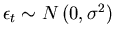.

A more complex model may be used, such as the standard multiplicative economic model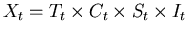;,

which is estimated in business statistics texts by the standard decomposition process. It is only in introductory time series study that the model approach is typically not explicit; intermediate and advanced courses deal most thoroughly with model identification and selection.

## 5.3 Inference for Cross-Sectional Data

59 For cross-sectional data collected on a sample, the probability model for the population is inferred from the sample results. At the simplest level, the sample data are taken as the model. This is commonly done, for example, in newspaper reports of surveys. It is also done in textbooks on introductory probability using the 'frequentist' approach, particularly for examples using simple contingency tables. It is rarely made clear that the process of inference is involved.

60 Introductory formal statistical inference typically deals with means and proportions. There are good practical reasons for this: these are the parameters of the population in which the analyst is most commonly interested. Not surprisingly, they coincide with the 'best prediction' parameters.

61 For a nominal variable, since the best prediction is the mode, the probability of each outcome must be estimated. Under random selection, this probability is the population proportion for that outcome, and it is estimated by the corresponding sample proportion.

62 For a numerical variable, since the best prediction is the mean, this has to be estimated. As is well known, the best estimator for the population mean on the criteria of unbiasedness and minimum variance (and as maximum likelihood estimator) is the sample mean. These criteria correspond to those for 'best prediction.' This estimates the population model mean, rather than the 'true' population mean, although if the population is very clearly defined and known the two will be identical.

63 The model is again the constant mean model. If only the prediction is required, it is sufficient to estimate the mean, so the simplest model is sufficient:;iid.

However, to obtain a confidence interval, for a large sample the model is specified as;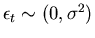iid

or;iid.

64 The central limit theorem tells us that a good model for the sampling distribution of the standardised sample mean of a simple random sample from a large population is the standard normal, provided n is large enough. How large is 'large enough' depends on how appropriate the normal model is for X itself. In practice this theorem is always required, since the normal distribution is a model, so strictly X is never 'normally distributed.' Similarly, if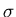is not known, but normality is a good model for X, then a t distribution is a good model for the standardised mean.

65 Using, for example, the t model, a two-sided (1-)% confidence interval for the population model mean has limits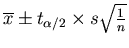.

## 5.4 Prediction for Cross-Sectional Sample Data

66 Based on a set of sample data, what is the best predictor? For a nominal variable, the best prediction is the mode, the value with the highest probability of occurrence. Since the corresponding sample proportion is the best estimate of this probability, the sample mode is the best predictor. The probability of error is estimated by the corresponding proportion.

67 For a numeric variable, the sample mean best estimates the model mean, which in turn is the best predictor, under both the criteria of zero absolute expected error and minimum expected squared error. Any sample-based candidate for the best predictor can be tested against the sample, calculating the absolute mean error and mean squared error. This process parallels that for the identification of the best prediction, where the predictors are tested against the population. Under this test, the sample mean performs best, again giving zero absolute mean error and minimum mean squared error.

68 To obtain a prediction interval for this forecast, the uncertainty in the estimate of the mean is combined with the variability assumed in the model for X. Using the t model, for example, a two-sided (1-)% prediction interval has limits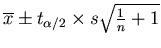.

The calculation of the variance for the prediction interval combines the variance of the probability model and that of the sampling distribution by using the Pythagorean Theorem.

# 6. Concluding Remarks

69 It has been argued in this paper that the underlying purpose, often implicit rather than explicit, of every statistical analysis is to predict values of one or more variables, based on probability models for the variables, to enable decisions. The models are in turn based on sample data. The 'best prediction' for a nominal variable based on the chosen model is the mode; the quality of the prediction is measured by the probability of error. If the prediction is based on a random sample, the best predictor is the sample mode. For a numeric variable the 'best prediction' based on the chosen model is the model mean; the quality of the prediction can be specified using a prediction interval. If the prediction is based on a random sample, the best predictor is the sample mean. Note that in each case, the sample statistic is used as an estimator for the model parameter, and as a predictor for values of the variable.

70 The usefulness of a statistical analysis depends on the quality of the predictions to which it leads: if a statistical analysis leads to useful forecasts, it is itself useful. An analysis that does not lead to useful predictions, however mathematically elegant, is of no practical use, except in the case when it shows that useful forecasts cannot be obtained.

71 This view of what statistics 'is' provides a powerful unifying approach to teaching the subject. If it is accepted that this view of the underlying thrust of statistics is correct, then it is reasonable that texts should reflect this view. The predictive use of probability models, including the use of prediction intervals, should be emphasised. And, of utmost importance, the practical usefulness of results must be emphasised.

## Acknowledgments

The author would like to thank the reviewers and editor for their help in developing this paper. A very early version of this material appeared in McLean (1998).

# References

Bhattacharyya, G. K., and Johnson, R. A. (1977), Statistical Concepts and Methods, New York: Wiley.

de Finetti B. (1972), Probability, Induction and Statistics, Chichester: Wiley.

DeGroot, M. H. (1986), "A Conversation With Persi Diaconis," Statistical Science, 1(3), 319-334.

Deming, W. E. (1950), Some Theory of Sampling, New York: Dover.

Foddy, W. H. (1988), Elementary Applied Statistics for the Social Sciences, Sydney: Harper & Row.

Freeman, L. C. (1965), Elementary Applied Statistics, New York: Wiley.

Hoel, P. G. (1971), Introduction to Mathematical Statistics (4th ed.), New York: Wiley.

Laplace, P. S. (Marquis de) (1814), A Philosophical Essay on Probabilities, trans. F. W. Truscott and F. L. Emory (1995 ed.), New York: Dover.

Levine, D. M., Berenson, M. L., Stephan, D. (1997), Statistics for Managers Using Microsoft Excel, Upper Saddle River, NJ: Prentice-Hall.

McLean, A. L. (1998), "The Forecasting Voice: A Unified Approach to Teaching Statistics," in Proceedings of the Fifth International Conference on Teaching of Statistics, Vol. 3, eds. L. Pereira-Mendoza, L. S. Kea, T. W. Kee, and W.-K. Wong, The Netherlands: International Statistical Institute, pp. 1193-1199.

Selvanathan, A., Selvanathan, S., Keller, G., Warrack, B., Bartel, H. (1994), Australian Business Statistics, Melbourne: Nelson.

Shavelson, R. J. (1981), Statistical Reasoning for the Behavioural Sciences, Boston: Allyn & Bacon.

Alan McLean
Department of Econometrics and Business Statistics
Monash University